This is the note I used as an example of applications in Linear Algebra I lectured at Purdue University. It is slightly modified so that it is more or less self contained.

Starting from least-squares solution, we are going to give an introductory exploration on (linear) regression in this note.

import numpy as np
import sklearn.linear_model
import matplotlib.pyplot as plt
from IPython.display import set_matplotlib_formats

plt.rcParams["figure.figsize"] = (8, 6)
set_matplotlib_formats('png', 'pdf')


## Least-squares solution

Let $A$ be an $m \times n$ matrix, and $B$ be a vector in $\mathbb{R}^m$. A least-squares solution to a linear system $Ax = B$ is an $\hat{x}$ such that $|A \hat{x} - B| \le |A x - B|$ for all $x$. Here, $|x|$ is the length of the vector $x$. If the system $Ax = B$ is consistent, then a least-squares solution is just a solution.

We have two ideas to get a consistent system out of $Ax = B$ in order to solve for $\hat{x}$:

• Using projection: $Ax = \mathrm{Proj}_{\mathrm{Col}A} B$, where $\mathrm{Col} A$ is the column space of $A$ spanned by columns of $A$.
• Using normal equation: $A^t Ax = A^t B$, where $A^t$ is the transpose of $A$.

The normal equation is the one we usually use. If columns of $A$ are linearly independent, then $A^tA$ is an invertible matrix. In this case, there is a unique least-squares solution

$$\hat{x} = (A^tA)^{-1}A^tB.$$

Least-squares solutions provide us a tool for regression analysis, including both linear or non-linear regression.

Example. Below is a table of monthly production cost according to production output for a hosiery mill. Production cost is in \$1000, and production output is in thousands of dozens of pairs. (Data source: http://users.stat.ufl.edu/~winner/data/millcost.txt)

    Output (O)    13.11      14.35     17.09      20.25      24.58      28.20
Cost (C)      29.45      31.40     38.01      43.65      50.25      56.09


Find a linear relation between the production cost and the production output.

We will first plot all data points to verify that they are in a linear relation.

output = np.array([
13.11, 14.35, 17.09, 20.25, 24.58, 28.20
])
cost = np.array([
29.45, 31.40, 38.01, 43.65, 50.25, 56.09
])
plt.plot(output, cost, 'ro')
plt.xlabel("production output", fontsize=18)
plt.ylabel("production cost", fontsize=18)
plt.title("Cost vs Output", fontsize=18)
plt.show()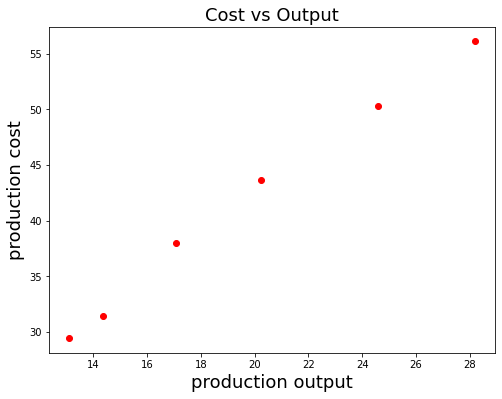Suppose $C = a O + b$, where $C$ is the cost, $O$ is the output, $a$ and $b$ are parameters needed to be determined. If we assume that the linear relation is perfect, then from the table of data, we get a system of linear equations in $a$ and $b$:

\begin{align*} 13.11 a + b &= 29.45 \\ 14.35 a + b &= 31.40 \\ 17.09 a + b &= 38.01 \\ 20.25 a + b &= 43.65 \\ 24.58 a + b &= 50.25 \\ 28.20 a + b &= 56.09 \end{align*}

However, from the figure above, we see data points are almost on the same line, but they are not exactly on that line. So the linear relation is not perfect, and the above system in $a$ and $b$ is not consistent. We want to find the best $a$ and $b$ for this inconsistent system, and that is how least-squares solutions come into sight. We write the system in the matrix form $Ax = B$, and use the normal equation to solve for $\hat{x}$. In this example, $A$ has linear independent columns, so it has a unique least-square solution

$$\hat{x} = (A^tA)^{-1} A^tB.$$
A = np.array([output, [1 for _ in range(6)]]).transpose()
A

array([[13.11,  1.  ],
[14.35,  1.  ],
[17.09,  1.  ],
[20.25,  1.  ],
[24.58,  1.  ],
[28.2 ,  1.  ]])
B = cost.reshape(-1, 1)
B

array([[29.45],
[31.4 ],
[38.01],
[43.65],
[50.25],
[56.09]])
xhat = np.linalg.inv(A.transpose() @ A) @ A.transpose() @ B
xhat

array([[1.7722402 ],
[6.74499953]])
a, b = xhat.flatten()
print(f"We get a = {a:.2f}, b={b:.2f}, so C = {a:.2f} O + {b:.2f}.")

We get a = 1.77, b=6.74, so C = 1.77 O + 6.74.

O = np.linspace(12, 30, 100)
C = a * O + b

plt.plot(output, cost, 'ro', label="Truth")
plt.plot(O, C, 'b', label="Prediction")
plt.xlabel("production output", fontsize=18)
plt.ylabel("production cost", fontsize=18)
plt.title("Cost vs Output", fontsize=18)
plt.legend()
plt.show()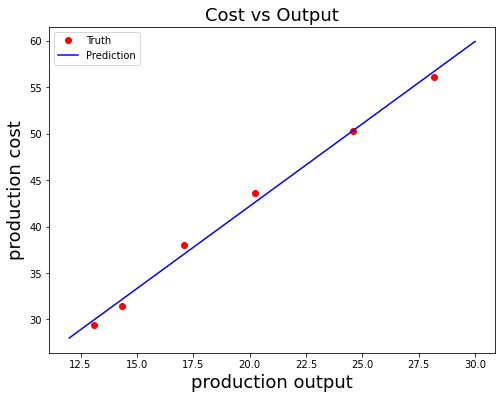The least-squares technique works in other kinds of regression problems as well. Let's take a look at the following example for polynomial regression.

Example. In an agricultural study of Fertilizer-Yield Relationship, we have a table of pounds of nitrogen used (lbs/acre) and yield (bushels) as follow. (Data source: http://users.stat.ufl.edu/~winner/data/fertyld.txt)

Nitrogen/acre  (in 20 lbs)           Yield (bushels)
0                            24.5
1                            41.5
2                            52.1
3                            61.4
4                            73.5
6                            86.0
8                            92.8
9                            94.0


Fit Yield as a second degree polynomial in Nitrogen/acre, i.e., find parameters $a, b$ and $c$ such that $Y = a + bN + cN^2$ fits the data the best, where $Y$ is yield in bushels and $N$ is Nitrogen/acre in the unit of 20 lbs.

nitrogen = np.array([
0, 1, 2, 3, 4, 6, 8, 9
])
yield_ = np.array([
24.5, 41.5, 52.1, 61.4, 73.5, 86.0, 92.8, 94.0
])
plt.plot(nitrogen, yield_, 'ro')
plt.xlabel("Nitrogen/acre", fontsize=18)
plt.ylabel("Yield", fontsize=18)
plt.title("Fertilizer-Yield Relationship", fontsize=18)
plt.show()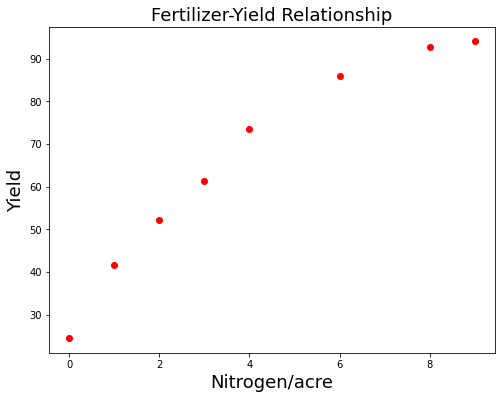Using $Y = a + b N + c N^2$ to fit our data, we expect to get

\begin{align*} a &= 24.5 \\ a + b + c &= 41.5 \\ a + 2b + 4c &= 52.1 \\ a + 3b + 9c &= 61.4 \\ a + 4b + 16c &= 73.5 \\ a + 6b + 36c &= 86.0 \\ a + 8b + 64c &= 92.8 \\ a + 9b + 81c &= 94.0 \end{align*}

We need to find the least-squares solution for this system to solve for $a, b$ and $c$.

A = np.array([
[1 for _ in range(len(nitrogen))], nitrogen, nitrogen * nitrogen
]).transpose()
A

array([[ 1,  0,  0],
[ 1,  1,  1],
[ 1,  2,  4],
[ 1,  3,  9],
[ 1,  4, 16],
[ 1,  6, 36],
[ 1,  8, 64],
[ 1,  9, 81]])
B = yield_.reshape(-1, 1)
B

array([[24.5],
[41.5],
[52.1],
[61.4],
[73.5],
[86. ],
[92.8],
[94. ]])
xhat = np.linalg.inv(A.transpose() @ A) @ A.transpose() @ B
xhat

array([[25.3953155 ],
[15.08787638],
[-0.8306277 ]])
a, b, c = xhat.flatten()
print(f"Y = {a:.2f} + {b:.2f}N + ({c:.2f})N^2.")

Y = 25.40 + 15.09N + (-0.83)N^2.

N = np.linspace(0, 10, 100)
Y = a + b * N + c * N * N
plt.plot(nitrogen, yield_, 'ro', label="Truth")
plt.plot(N, Y, 'b', label="Prediction")
plt.xlabel("Nitrogen/acre", fontsize=18)
plt.ylabel("Yield", fontsize=18)
plt.title("Fertilizer-Yield Relationship", fontsize=18)
plt.legend()
plt.show()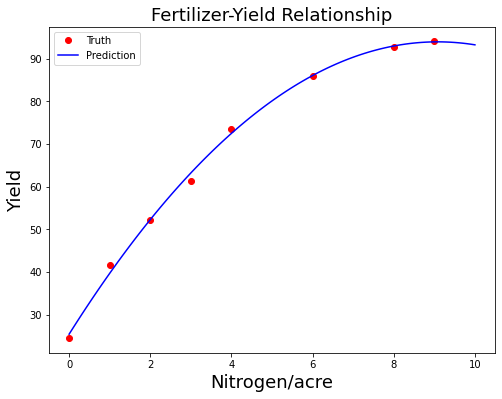## Squared error loss function

The least-squares technique is powerful for regression analysis. However, it is not in its most general form. More importantly, it is not memory-efficient and computation-efficient. We are going to generalize the least-squares technique using the squared error loss function.

Suppose we have a set of observed data $\{(x_i, y_i): i = 1, 2, ..., t\}$, where $x_i \in \mathbb{R}^n$ and $y_i \in \mathbb{R}^m$. The task of regression analysis is to find a mathematical function $f: \mathbb{R}^n \to \mathbb{R}^m$ (model) such that $y = f(x)$ fit the observed data the best. Here, $x \in \mathbb{R}^n$ is the independent variable (features) and $y \in \mathbb{R}^m$ is the dependent variable (targets). To quantify the quality of the model $f$, we can use the squared error loss function

$$L(f) = \sum_{i = 1}^t |f(x_i) - y_i|^2.$$

The smaller the loss is, the better the model is. Therefore, to find the best model is to find the model $\hat{f}$ that minimizes $L(\hat{f})$. It turns out that minimzing the squared error loss function to find the best model is a generalization of finding the least-squares solution of certain linear systems.

Techniques to minimizing the squared error loss function $L$ are deeply related to (partial) derivatives in calculus. One way to minimize $L$ is to set all partial derivatives equal 0 to get a linear system, whihc we solve to get $\hat{f}$. Another way to minimize $L$ is by gradient descent, which utilizes the fact that the gradient of a function points towards the direction of fastest increase while the negative of the gradient points towards the direction of fastest decrease.

Example. We redo the previous example using the squared error loss function. The model $f: \mathbb{R} \to \mathbb{R}$ we want is a second degree polynomial

$$f(x) = a + bx + cx^2,$$

with parameters $a, b$ and $c$. The squared error loss function in this example is

\begin{align*} L(a, b, c) &= (a - 24.5)^2 + (a + b + c - 41.5)^2 + (a + 2b + 4c - 52.1)^2 \\ &+ (a + 3b + 9c - 61.4)^2 + (a + 4b + 16c - 73.5)^2 + (a + 6b + 36c - 86.0)^2\\ &+ (a + 8b + 64c - 92.8)^2 + (a + 9b + 81c - 94.0)^2. \end{align*}

Minimizing this loss function using techniques in calculus, we also get

$$a = 25.40, b = 15.09, c = -0.83.$$

## Linear Model

A linear model from a feature space $\mathbb{R}^n$ to a target space $\mathbb{R}^m$ is a map $T: \mathbb{R}^n \to \mathbb{R}^m$ by

$$T(x) = Ax + B,$$

where $A$ is an $m \times n$ matrix and $B \in \mathbb{R}^m$. We say $A$ and $B$ are parameters for the model $T$. If we have observed data $\left\{(x_i, y_i): i = 1, 2, ..., t\right\}$, then $A$ and $B$ can be found by minimizing the squared error loss function

$$L(A, B) = \sum_{i=1}^t |Ax_i + B - y_i|^2.$$

Example. We apply linear model in the task of handwritten-digits recognition, in which we used the famous MNIST dataset. You can download the dataset on http://yann.lecun.com/exdb/mnist/. Let's take a look at some samples of the MNIST dataset.

with open('train-labels.idx1-ubyte', 'rb') as lbpath:
train_labels = np.frombuffer(lbpath.read(), dtype=np.uint8, offset=8)
with open('train-images.idx3-ubyte', 'rb') as imgpath:
train_images = np.frombuffer(imgpath.read(), dtype=np.uint8,
offset=16).reshape(-1, 784)

r = c = 5
fig, ax = plt.subplots(r, c)
for i in range(r):
for j in range(c):
k = i * c + j
img = train_images[k]
img = img.reshape((28, 28))
lbl = train_labels[k]
ax[i, j].imshow(img, cmap='gray_r')
ax[i, j].set_title(lbl)
ax[i, j].axis('off')
plt.show()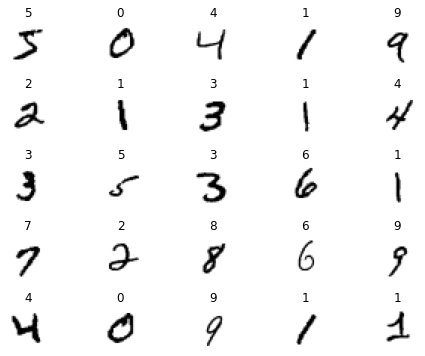If we look at one image, it is of size $28 \times 28$. We can treat it as a $28 \times 28$ matrix, or treat it as a vector in $\mathbb{R}^{28 \times 28} = \mathbb{R}^{784}$. So our inputs $x$ are vectors of size $784$. On the other hand, to represent the digits 0, 1, ..., 9, we use vectors in $\mathbb{R}^{10}$. For example, digit 0 is represented by $\begin{bmatrix} 1 & 0 & 0 & 0 & 0 & 0 & 0 & 0 & 0 & 0\end{bmatrix} ^t$, and digit 9 is represented by $\begin{bmatrix} 0 & 0 & 0 & 0 & 0 & 0 & 0 & 0 & 0 & 1\end{bmatrix} ^t$
. So our targets $y$ are vectors of size $10$. Our task is to find a linear model $T: \mathbb{R}^{784} \to \mathbb{R}^{10}$ that fits the data the best.

x = train_images
y = np.zeros((train_labels.size, 10))
y[np.arange(train_labels.size), train_labels] = 1
model = sklearn.linear_model.LinearRegression().fit(x, y)


If we write $T = Ax + B$, then $A$ is a $10 \times 784$ matrix and $B \in \mathbb{R}^{10}$.

print(f"A is of the shape: {model.coef_.shape}")
print(f"B is of the shape: {model.intercept_.shape}")

A is of the shape: (10, 784)
B is of the shape: (10,)


Given an vector in $\mathbb{R}^{784}$, the model will produce a vector in $\mathbb{R}^{10}$. We might think of the resulting vector as a collection of scores of all digits for the given image.

model.predict(x[])

array([[ 0.08495223,  0.00138597, -0.09322946,  0.43078067, -0.2069839 ,
0.5104715 ,  0.18358026,  0.31422564, -0.12952326, -0.10211847]])

Digit 5 has the highest score 0.51 in the prediction, so the model predict that the first image is a digit 5, which is correct. Let's see the performance of the model.

predicted_labels = np.argmax(model.predict(x), axis=1)
train_accuracy = np.sum(predicted_labels == train_labels) / train_labels.size
print(f"Train accuracy: {train_accuracy * 100:.2f}%")

Train accuracy: 85.78%

with open('t10k-labels.idx1-ubyte', 'rb') as lbpath:
test_labels = np.frombuffer(lbpath.read(), dtype=np.uint8, offset=8)
with open('t10k-images.idx3-ubyte', 'rb') as imgpath:
test_images = np.frombuffer(imgpath.read(), dtype=np.uint8,
offset=16).reshape(-1, 784)
predicted_labels = np.argmax(model.predict(test_images), axis=1)
test_accuracy = np.sum(predicted_labels == test_labels) / test_labels.size
print(f"Test accuracy: {test_accuracy * 100:.2f}%")

Test accuracy: 86.03%


Using a simple linear model gets a reasonable performance for digits recognition. We can use linear model as a building block, pairing with other deep learning techniques, to build a far better model. The state of the art model can achieve 99.77% accuracy on the test set.